# CPM Statistics Resources

### Web-based eTools, organized by type

The tools below are designed to help you explore various concepts of statistics. Tools on this page are general-use and could potentially be used by any statistics teacher or student.

Looking for Desmos activities? Try the teacher resources page.

# Exploring and Graphing Data eTools

### Univariate Data Explorer and Grapher

#### Histograms, dot plots, boxplots, summary statistics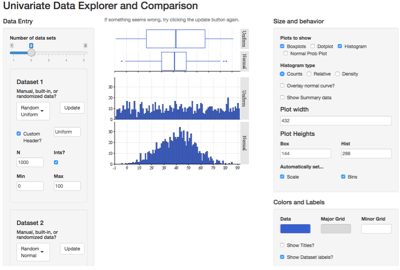Create histograms, boxplots, dot plots, and normal probability plots for up to five quantitative variables on the same axis for easy comparison. Allows for customization of graph appearance. Enter data manually, use preloaded data from the text, or generate random data using a variety of distributions.

### Bivariate Data Explorer and Grapher

#### Scatterplots and linear regression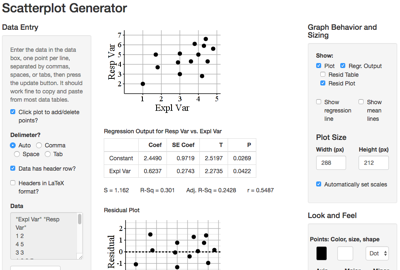Create customizable scatterplots and residual plots from bivariate data. Perform linear regression on the data. Data can bye typed, copied-and-pasted from spreadsheets, randomly generate points, or added to the graph by clicking.

# Simulations and Inference eTools

### Proportion Sampling Distribution Simulator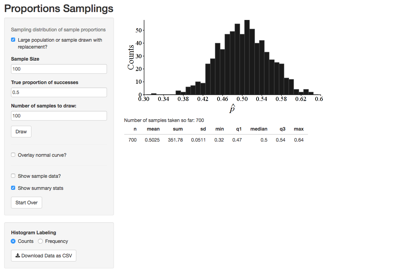Generate simulated sample proportions from a population of either infinite or known finite size to explore the sampling distribution of the sample proportion.

### Proportion Confidence Interval Simulator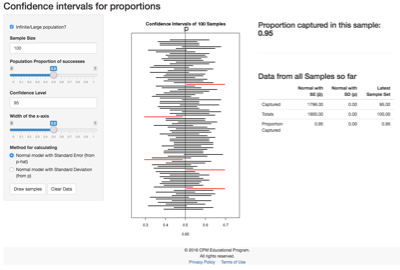Simulate many confidence intervals for a population proportion using a variety of techniques and parameters and explore the capture rate of the confidence intervals over time.

### Quantitative Sampling Distribution Simulator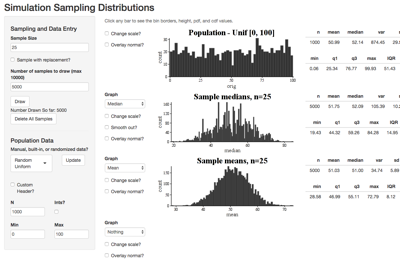Generate and display sampling distributions of many different quantiative statistics (or even make your own!) from a population of your choice, including a variety of randomly generated options.

### Mean Confidence Interval Simulator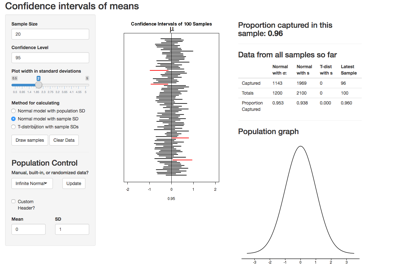Simulate confidence intervals for a population mean using a wide variety of techniques, from a population of your choice. Explore how different populations and interval techniques affect the capture rate and interval width.

### Errors and Power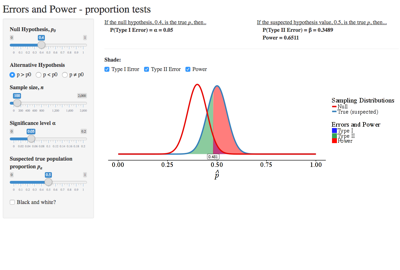Show the relationship between the "true" sampling distribution and the "hypothesis" sampling distribution for proportion hypothesis tests, and use that to explore the likelihood of Type I and Type II errors and the power of the test.

# Statistical Distribution eTools

### Binomial Distribution Explorer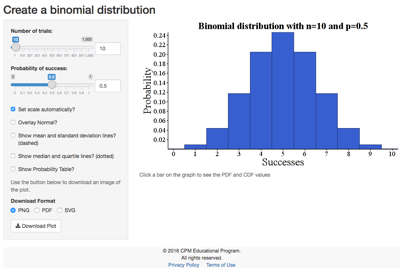Graph binomial distributions, click for probabilities, and overlay the normal curve.

### Normal Distribution Explorer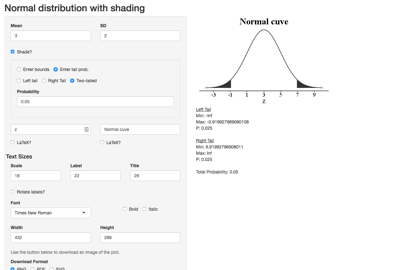Graph custom, optionally shaded, normal distributions. Find areas under the curve and critical values.

### t-Distribution Explorer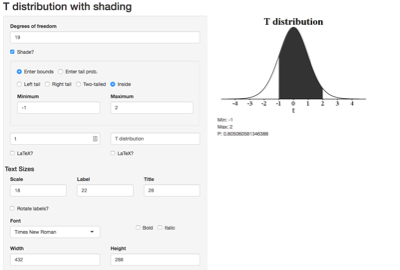Graph custom, optionally shaded, Students' t-distributions. Find areas under the curve and critical values.

### χ2 Distribution Explorer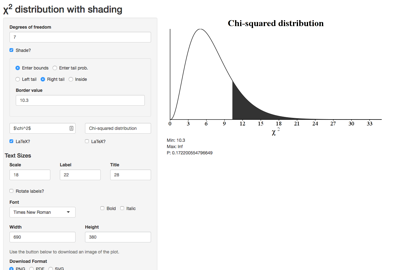Graph custom, optionally shaded, chi-squared distributions. Find areas under the curve and critical values.

### F-Distribution Explorer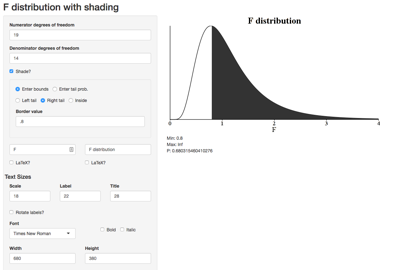Graph custom, optionally shaded, F-distributions. Find areas under the curve and critical values.

# Activities, Demonstrations, and Experiments

### Reaction Time Experiment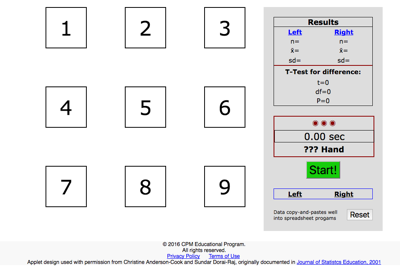A two-part activity to explore several properties of experimental design. Associated with Lessons 4.2.3 and 11.1.1 of the CPM AP Statistics book.

### Blocking Experiment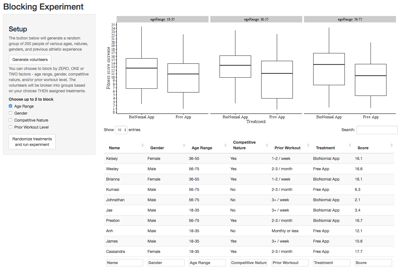This applet allows simulation of an imaginary experiment involving two exercise tracking apps. By experimenting with different blocking variables, you can see how clever blocking can greatly reduce the variability in an experiment and make results easier to see. Associated with Lesson 4.2.2 of the CPM AP Statistics book.

### t-Distribution Animation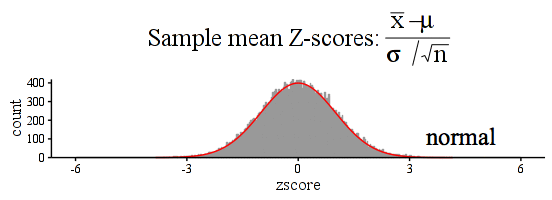Observe how the t-distribution changes based on the sample size! (Click for a larger version)